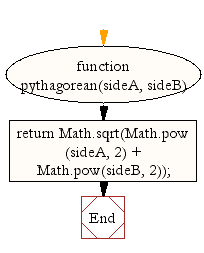# JavaScript: The Pythagorean theorem

## JavaScript Math: Exercise-35 with Solution

Write a JavaScript function for the Pythagorean theorem.

According to Wikipedia : In mathematics, the Pythagorean theorem, also known as Pythagoras' theorem, is a relation in Euclidean geometry among the three sides of a right triangle. It states that the square of the hypotenuse (the side opposite the right angle) is equal to the sum of the squares of the other two sides. The theorem can be written as an equation relating the lengths of the sides a, b and c, often called the "Pythagorean equation".

Test Data :
console.log(pythagorean(4, 3));
5

Sample Solution:-

HTML Code:

``````<!DOCTYPE html>
<html>
<meta charset="utf-8">
<title>Write a JavaScript function for the Pythagorean theorem</title>
<body>

</body>
</html>
```
```

JavaScript Code:

``````function pythagorean(sideA, sideB){
return Math.sqrt(Math.pow(sideA, 2) + Math.pow(sideB, 2));
}

console.log(pythagorean(4, 3));
```
```

Sample Output:

```5
```

Pictorial Presentation:Flowchart:Live Demo:

See the Pen javascript-math-exercise-35 by w3resource (@w3resource) on CodePen.

Improve this sample solution and post your code through Disqus

What is the difficulty level of this exercise?

﻿

## JavaScript: Tips of the Day

Capitalizes the first letter of a string

Example:

```const tips_capitalize = ([first, ...rest], lowerRest = false) =>
first.toUpperCase() + (lowerRest ? rest.join('').toLowerCase() : rest.join(''));

console.log(tips_capitalize('javaScript'));
console.log(tips_capitalize('javaScript', true));
```

Output:

```JavaScript
Javascript
```